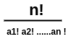Home Practice Programming Print all unique permutations of a string with duplicate characters

# Print all unique permutations of a string with duplicate characters

A permutation is an act of rearranging or reordering elements of a set or string uniquely etc.
For n elements, n! (n factorial) permutations are possible if there is no repetition.
For n elements,permutations are possible, where ak represents the occurrence of the kth character in the string.

Ex-> Possible permutations of aabc is = 4!/2! = 12.
abac abca aabc aacb acab acba baac baca bcaa cbaa caba caab

Before solving this question, please refer to this question.

#### Algorithm

Everything is the same just like this question, except now we can have duplicates too. To avoid duplication we have to make sure that we don’t repeat the selection of a character.

We have to check before considering a character whether it is repeated or not.

```can_swap(str, start, i){
for(j=start; j<i; j++){
if(str[j]==str[i]
return false;
return true;
}

find_permutation(str, start, end){
if(start == end)
print(str)
for(i=start; i<end; i++){
if(can_swap(str, start,i)==true){
swap(str[start], str[i]);
//we fixed the ith index and now in next recursion
//we will work with the remaining string elements
find_permutation(str, start+1, end);
swap(str[i], str[start]);
}
}
return 0;
}```

#### Implementation of the above algorithm in CPP

```#include <bits/stdc++.h>
using namespace std;

bool can_swap(string str, int start, int i){

for(int j=start;j<i;j++){
if(str[i]==str[j])
return false;
}
return true;
}

int find_permutation(string str, int start, int end){

if(start==end)
cout<<str<<endl;

for(int i=start; i<end; i++){

if(can_swap(str, start, i)){

swap(str[start],str[i]);
find_permutation(str, start+1,end);
swap(str[start],str[i]);
}
}
return 0;
}

int main()
{
string str = "abac";
find_permutation(str, 0, str.size());

return 0;
}
```

```abac
abca
aabc
aacb
acab
acba
baac
baca
bcaa
cbaa
caba
caab```